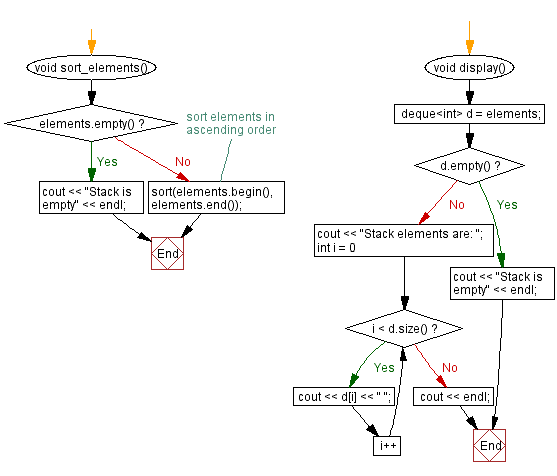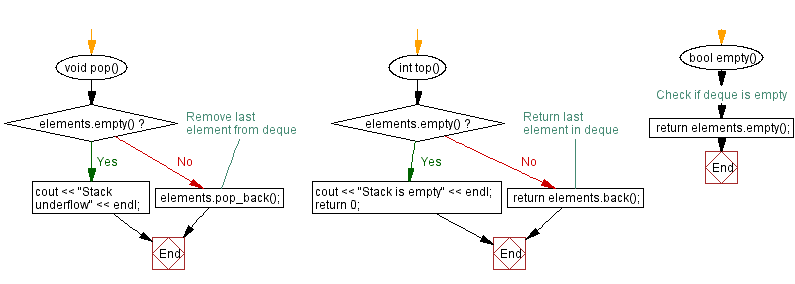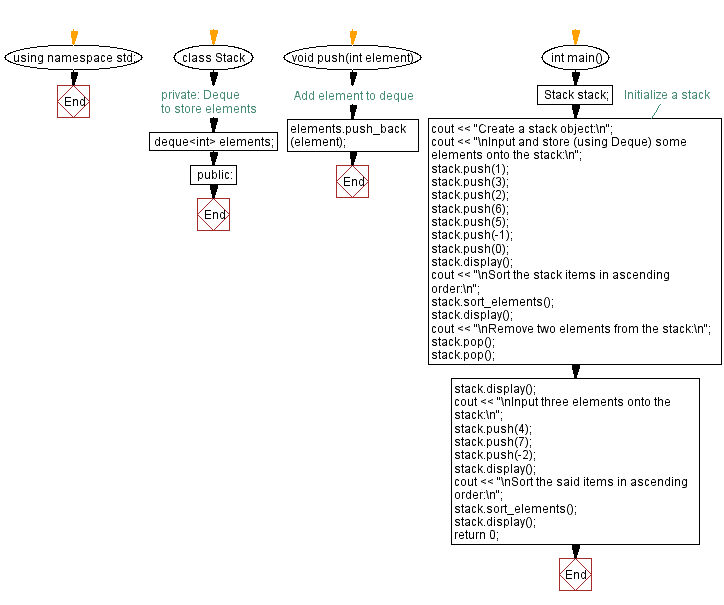﻿ C++ Sort the stack (using a Deque) elements

# C++ Stack Exercises: Sort the stack (using a Deque) elements

## C++ Stack: Exercise-29 with Solution

Write a C++ program that sorts the stack (using a Deque) elements.

Test Data:
Create a stack object:
Input and store (using Deque) some elements onto the stack:
Stack elements are: 1 3 2 6 5 -1 0
Sort the stack items in ascending order:
Stack elements are: -1 0 1 2 3 5 6

Sample Solution:

C++ Code:

``````#include <iostream>
#include <deque>
#include<algorithm>

using namespace std;

class Stack {
private:
deque<int> elements; // Deque to store elements

public:
void push(int element) {
elements.push_back(element); // Add element to deque
}

void pop() {
if (elements.empty()) {
cout << "Stack underflow" << endl;
} else {
elements.pop_back(); // Remove last element from deque
}
}

int top() {
if (elements.empty()) {
cout << "Stack is empty" << endl;
return 0;
} else {
return elements.back(); // Return last element in deque
}
}

bool empty() {
return elements.empty(); // Check if deque is empty
}

void sort_elements(){
if (elements.empty()) {
cout << "Stack is empty" << endl;
return;
}

// sort elements in ascending order
sort(elements.begin(), elements.end());
}

void display() {
deque<int> d = elements;
if (d.empty()) {
cout << "Stack is empty" << endl;
return;
}
cout << "Stack elements are: ";
for (int i = 0; i < d.size(); i++) {
cout << d[i] << " ";
}
cout << endl;
}

};

int main() {
Stack stack;
//Initialize a stack
cout << "Create a stack object:\n";
cout << "\nInput and store (using Deque) some elements onto the stack:\n";
stack.push(1);
stack.push(3);
stack.push(2);
stack.push(6);
stack.push(5);
stack.push(-1);
stack.push(0);
stack.display();
cout << "\nSort the stack items in ascending order:\n";
stack.sort_elements();
stack.display();
cout << "\nRemove two elements from the stack:\n";
stack.pop();
stack.pop();
stack.display();
cout << "\nInput three elements onto the stack:\n";
stack.push(4);
stack.push(7);
stack.push(-2);
stack.display();
cout << "\nSort the said items in ascending order:\n";
stack.sort_elements();
stack.display();
return 0;
}
``````

Sample Output:

```Create a stack object:

Input and store (using Deque) some elements onto the stack:
Stack elements are: 1 3 2 6 5 -1 0

Sort the stack items in ascending order:
Stack elements are: -1 0 1 2 3 5 6

Remove two elements from the stack:
Stack elements are: -1 0 1 2 3

Input three elements onto the stack:
Stack elements are: -1 0 1 2 3 4 7 -2

Sort the said items in ascending order:
Stack elements are: -2 -1 0 1 2 3 4 7
```

Flowchart:CPP Code Editor: